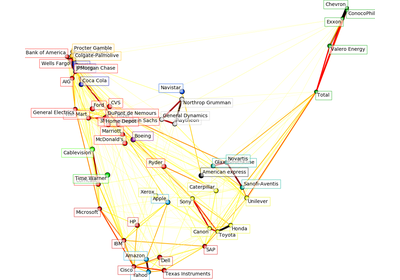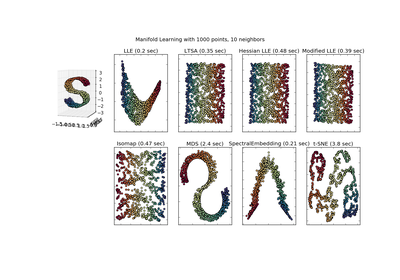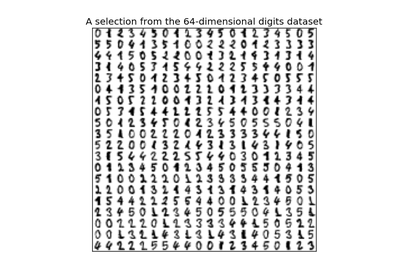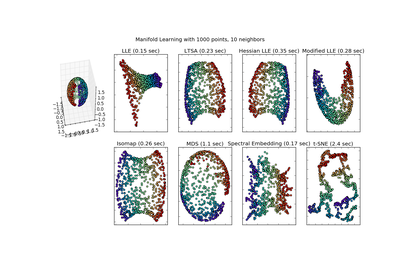# `sklearn.manifold`.LocallyLinearEmbedding¶

class `sklearn.manifold.``LocallyLinearEmbedding`(n_neighbors=5, n_components=2, reg=0.001, eigen_solver='auto', tol=1e-06, max_iter=100, method='standard', hessian_tol=0.0001, modified_tol=1e-12, neighbors_algorithm='auto', random_state=None, n_jobs=1)[source]

Locally Linear Embedding

Read more in the User Guide.

Parameters: n_neighbors : integer number of neighbors to consider for each point. n_components : integer number of coordinates for the manifold reg : float regularization constant, multiplies the trace of the local covariance matrix of the distances. eigen_solver : string, {‘auto’, ‘arpack’, ‘dense’} auto : algorithm will attempt to choose the best method for input data arpack : use arnoldi iteration in shift-invert mode.For this method, M may be a dense matrix, sparse matrix, or general linear operator. Warning: ARPACK can be unstable for some problems. It is best to try several random seeds in order to check results. dense : use standard dense matrix operations for the eigenvaluedecomposition. For this method, M must be an array or matrix type. This method should be avoided for large problems. tol : float, optional Tolerance for ‘arpack’ method Not used if eigen_solver==’dense’. max_iter : integer maximum number of iterations for the arpack solver. Not used if eigen_solver==’dense’. method : string (‘standard’, ‘hessian’, ‘modified’ or ‘ltsa’) standard : use the standard locally linear embedding algorithm. seereference  hessian : use the Hessian eigenmap method. This method requires`n_neighbors > n_components * (1 + (n_components + 1) / 2` see reference  modified : use the modified locally linear embedding algorithm.see reference  ltsa : use local tangent space alignment algorithmsee reference  hessian_tol : float, optional Tolerance for Hessian eigenmapping method. Only used if `method == 'hessian'` modified_tol : float, optional Tolerance for modified LLE method. Only used if `method == 'modified'` neighbors_algorithm : string [‘auto’|’brute’|’kd_tree’|’ball_tree’] algorithm to use for nearest neighbors search, passed to neighbors.NearestNeighbors instance random_state: numpy.RandomState or int, optional : The generator or seed used to determine the starting vector for arpack iterations. Defaults to numpy.random. n_jobs : int, optional (default = 1) The number of parallel jobs to run. If `-1`, then the number of jobs is set to the number of CPU cores. embedding_vectors_ : array-like, shape [n_components, n_samples] Stores the embedding vectors reconstruction_error_ : float Reconstruction error associated with embedding_vectors_ nbrs_ : NearestNeighbors object Stores nearest neighbors instance, including BallTree or KDtree if applicable.

References

 [R186] Roweis, S. & Saul, L. Nonlinear dimensionality reduction by locally linear embedding. Science 290:2323 (2000).
 [R187] Donoho, D. & Grimes, C. Hessian eigenmaps: Locally linear embedding techniques for high-dimensional data. Proc Natl Acad Sci U S A. 100:5591 (2003).
 [R188] Zhang, Z. & Wang, J. MLLE: Modified Locally Linear Embedding Using Multiple Weights. http://citeseerx.ist.psu.edu/viewdoc/summary?doi=10.1.1.70.382
 [R189] Zhang, Z. & Zha, H. Principal manifolds and nonlinear dimensionality reduction via tangent space alignment. Journal of Shanghai Univ. 8:406 (2004)

Methods

 `fit`(X[, y]) Compute the embedding vectors for data X `fit_transform`(X[, y]) Compute the embedding vectors for data X and transform X. `get_params`([deep]) Get parameters for this estimator. `set_params`(\*\*params) Set the parameters of this estimator. `transform`(X) Transform new points into embedding space.
`__init__`(n_neighbors=5, n_components=2, reg=0.001, eigen_solver='auto', tol=1e-06, max_iter=100, method='standard', hessian_tol=0.0001, modified_tol=1e-12, neighbors_algorithm='auto', random_state=None, n_jobs=1)[source]
`fit`(X, y=None)[source]

Compute the embedding vectors for data X

Parameters: X : array-like of shape [n_samples, n_features] training set. self : returns an instance of self.
`fit_transform`(X, y=None)[source]

Compute the embedding vectors for data X and transform X.

Parameters: X : array-like of shape [n_samples, n_features] training set. X_new: array-like, shape (n_samples, n_components) :
`get_params`(deep=True)[source]

Get parameters for this estimator.

Parameters: deep : boolean, optional If True, will return the parameters for this estimator and contained subobjects that are estimators. params : mapping of string to any Parameter names mapped to their values.
`set_params`(**params)[source]

Set the parameters of this estimator.

The method works on simple estimators as well as on nested objects (such as pipelines). The latter have parameters of the form `<component>__<parameter>` so that it’s possible to update each component of a nested object.

Returns: self :
`transform`(X)[source]

Transform new points into embedding space.

Parameters: X : array-like, shape = [n_samples, n_features] X_new : array, shape = [n_samples, n_components]

Notes

Because of scaling performed by this method, it is discouraged to use it together with methods that are not scale-invariant (like SVMs)

## Examples using `sklearn.manifold.LocallyLinearEmbedding`¶Visualizing the stock market structureComparison of Manifold Learning methodsManifold learning on handwritten digits: Locally Linear Embedding, Isomap...Manifold Learning methods on a severed sphere